#### 期刊菜单

Research on Open-Circuit Fault Diagnosis and Fault-Tolerant Control Strategy of Three-Phase Three-Level NPC Rectifier
DOI: 10.12677/AEPE.2021.95027, PDF, HTML, XML, 下载: 276  浏览: 448

Abstract: In recent years, the three-phase three-level NPC rectifier has been widely used in high voltage and high power fields. In order to improve the safety and reliability of NPC three-level rectifier opera-tion, it is particularly important to study its fault diagnosis and fault-tolerant control. In this paper, the changes of current flow path and fault characteristics of each switch device after open-circuit fault are analyzed in detail, and a fault diagnosis method based on current path is adopted. Then, the influence of the open-circuit fault of switch tube on space voltage vector is analyzed, and a fault-tolerant control strategy based on reference voltage vector correction is proposed. The fault detection method used in this paper can accurately locate the fault switch device, without a lot of calculation and rapid diagnosis. The proposed fault-tolerant control method does not need addi-tional hardware, which can effectively improve the reliability of NPC three-level rectifier. Finally, the effectiveness of the proposed method is verified by simulation.

1. 前言

2. 故障诊断方法

NPC型三电平整流器的主电路拓扑如图1所示。该整流器的每个桥臂由4个功率开关器件Sx1~Sx2 (x = a、b、c)和2个箝位二极管(VDx1、VDx2)组成，直流侧有两个电容C1、C2

NPC型三电平整流器每个桥臂可以输出P、O、N三种开关状态，三种开关状态分别对应输出Udc/2、0、−Udc/2三种电压(桥臂中点x到直流侧两电容中点n的电压)，见表1。开关状态P表示桥臂上面两个开关器件Sx1和Sx2同时导通，开关状态O表示开关器件Sx2和Sx3同时导通，开关状态N表示开关器件Sx3和Sx4同时导通。桥臂最上面的开关管Sx1和最下面的开关管Sx4被称为外部开关器件，中间的2个开关管Sx2和Sx3被称为内部开关器件。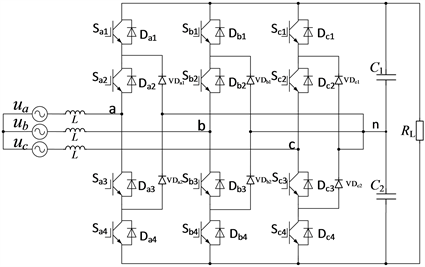Figure 1. Main circuit of NPC three-level rectifierTable 1. Output voltage and switching state

2.1. 外部开关器件故障诊断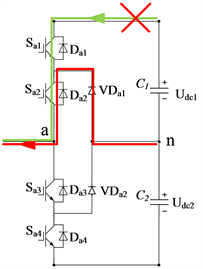(a)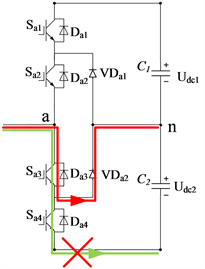(b)

Figure 2. Current path of external switch before and after open circuit: (a) Sa1 open circuit; (b) Sa4 open circuitTable 2. The judgment basis of open-circuit fault of external switching devices

2.2. 内部开关器件故障诊断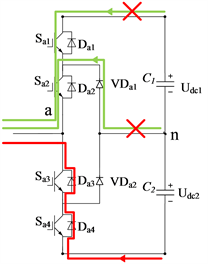(a)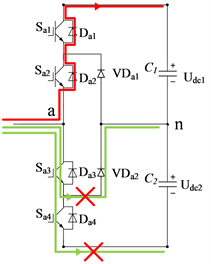(b)

Figure 3. Current path of internal switch before and after open circuit: (a) Sa2 open circuit; (b) Sa3 open circuitTable 3. The judgment basis of open-circuit fault of internal switching devices

3. 容错控制方法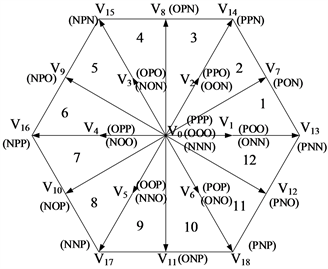Figure 4. 12 partitions of space voltage vector

NPC型三电平整流器的参考电压矢量可以利用SVPWM来实现。参考电压矢量V*可以由式(1)中的三个电压矢量组合而成。

${V}^{*}=\alpha {V}_{1}+\beta {V}_{2}+\gamma {V}_{n}+\gamma {V}_{p}\text{\hspace{0.17em}}\text{\hspace{0.17em}}\text{\hspace{0.17em}}\left(\alpha =\frac{{T}_{1}}{{T}_{s}},\beta =\frac{{T}_{2}}{{T}_{s}},\gamma =\frac{{T}_{0}}{2{T}_{s}}\right)$ (1)

${V}^{*}=\alpha {V}_{15}+\beta {V}_{9}+\gamma {V}_{3\text{n}}+\gamma {V}_{3p}$ (2)

${V}_{fault1}^{*}=\alpha {V}_{15}+\left(\beta +\gamma \right){V}_{9}+\gamma {V}_{3n}$ (3)

${V}_{comp1}^{*}=\alpha {V}_{15}+\beta {V}_{9}+2\gamma {V}_{3n}$ (4)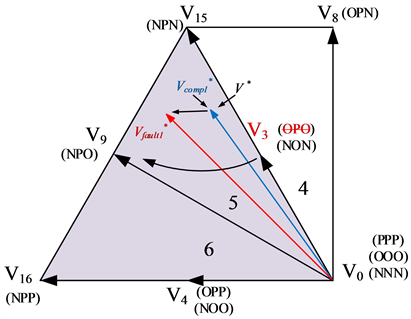(a)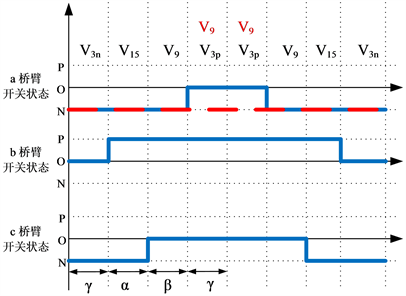(b)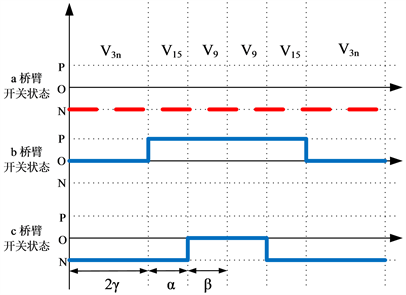(c)

Figure 5. Reference voltage vector and switching mode in case 1: (a) distortion and correction of reference voltage; (b) switching mode in fault condition; (c) fault-tolerant switching mode

${V}^{*}=\alpha {V}_{15}+\beta {V}_{8}+\gamma {V}_{3n}+\gamma {V}_{3p}$ (5)

${V}_{fault2}^{*}=\left(\alpha +\beta \right){V}_{15}+\gamma {V}_{3n}+\gamma {V}_{9}$ (6)

${V}_{comp2}^{*}=\left(\alpha +\beta /2\right){V}_{15}+\left(2\gamma +\beta /2\right){V}_{3n}$ (7)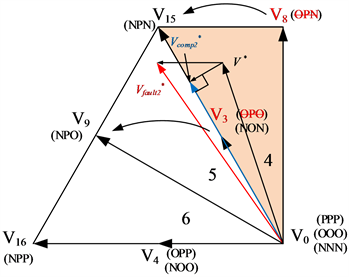(a)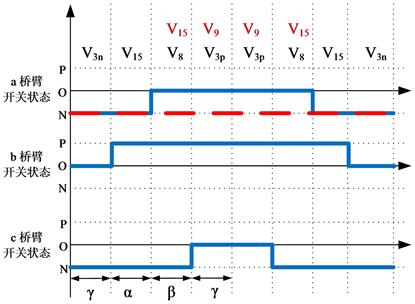(b)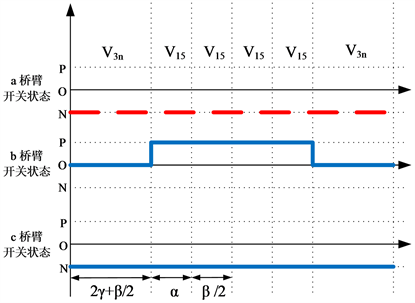(c)

Figure 6. Reference voltage vector and switching mode in case 2: (a) distortion and correction of reference voltage; (b) switching mode in fault condition; (c) fault-tolerant switching modeTable 4. Reference vectors for sectors 4, 5, 6

4. 仿真结果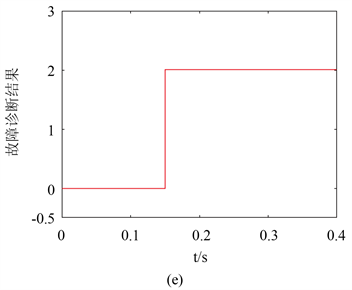Figure 7. Sa2 fault diagnosis: (a) a phase bridge arm switch state; (b) a phase current ia; (c) clamp diode current i_VDa1; (d) a phase arm voltage Uan; (e) fault diagnosis results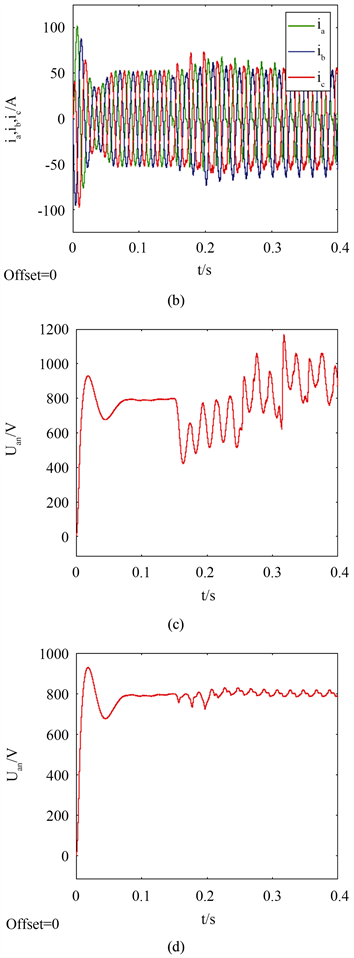Figure 8. AC side three-phase current and DC side voltage waveform: (a) three phase current waveform before and after fault; (b) three-phase current waveform before and after fault-tolerant control; (c) voltage waveform of DC side before and after fault; (d) voltage waveform of DC side before and after fault-tolerant controlTable 5. THD of three-phase current

5. 结论

NOTES

*通讯作者。

  金红元, 邹云屏, 林磊, 陈伟, 邹旭东, 钟和清, 等. 三电平PWM整流器双环控制技术及中点电压平衡控制技术的研究[J]. 中国电机工程学报, 2006, 26(20): 64-68.  Yang, S., Xiang, D., Bryant, A., Mawby, P., Ran, L. and Tavner, P. (2011) Condition Monitoring for Device Reliability in Power Electronic Converters: A Review. IEEE Trans-actions on Power Electronics, 25, 2734-2752. https://doi.org/10.1109/TPEL.2010.2049377  Li, X., Xu, D., Zhu, H., Cheng, X., Yu, Y. and Ng, W.T. (2018) An Indirect IGBT Over-Current Detection Technique via Gate Voltage Monitoring and Analyzing. IEEE Transactions on Power Electronics, 34, 3615-3622. https://doi.org/10.1109/TPEL.2018.2856777  Azer, P., Ouni, S. and Narimani, M. (2020) A Novel Fault-Tolerant Technique for Active Neutral Point Clamped Inverter Using Carrier-Based PWM. IEEE Transactions on Industrial Electronics, 67, 1792-1803. https://doi.org/10.1109/TIE.2019.2903764  Chen, Z.W., Ding, S.X., Peng, T., Yang, C. and Gui, W. (2018) Fault Detection for Non-Gaussian Processes Using Generalized Canonical Correlation Analysis and Randomized Algo-rithms. IEEE Transactions on Industrial Electronics, 65, 1559-1567. https://doi.org/10.1109/TIE.2017.2733501  Mendes, A.M.S., Abadi, M.B. and Cruz, S. (2014) Fault Diag-nostic Algorithm for Three-Level Neutral Point Clamped AC Motor Drives, Based on the Average Current Park’s Vector. IET Power Electronics, 7, 1127-1137. https://doi.org/10.1049/iet-pel.2013.0416  Sanchez, J.P., Campos-Delgado, D.U., Espinoza-Trejo, D.R., Val-dez-Fernández, A.A. and De Angelo, C.H. (2019) Fault Diagnosis in Grid-Connected PV NPC Inverters by a Mod-el-Based and Data Processing Combined Approach. IET Power Electronics, 12, 3254-3264. https://doi.org/10.1049/iet-pel.2018.5237  Ge, X., Pu, J., Gou, B. and Liu, Y.-C. (2018) An Open-Circuit Fault Diagnosis Approach for Single-Phase Three-Level Neutral-Point-Clamped Converters. IEEE Transactions on Power Electronics, 33, 2559-2570. https://doi.org/10.1109/TPEL.2017.2691804  Caseiro, L.M.A. and Mendes, A.M.S. (2015) Real-Time IGBT Open-Circuit Fault Diagnosis in Three-Level Neutral-Point-Clamped Voltage-Source Rectifiers Based on Instant Voltage Error. IEEE Transaction on Industrial Electronics, 62, 1669-1678. https://doi.org/10.1109/TIE.2014.2341558  Caseiro, L.M.A. and Mendes, A.M.S. (2015) Real-Time Multiple IGBT Open-Circuit Fault Diagnosis in Three-Level Neutral-Point-Clamped Converters with No Additional Sensors. IECON 2015-41st Annual Conference of the IEEE Industrial Electronics Society, Yokohama, 9-12 November 2015, 3181-3186. https://doi.org/10.1109/IECON.2015.7392590  Cheng, S., Chen, Y.T., Yu, T.J. and Wu, X. (2016) A Novel Diagnostic Technique for Open-Circuited Faults of Inverters Based on Output Line-to-Line Voltage Model. IEEE Transactions on Industrial Electronics, 63, 4412-4421. https://doi.org/10.1109/TIE.2016.2535960  许伟奇, 张斌, 汶雪. 基于分数阶滑模观测器的三相八开关容错逆变器驱动永磁同步电机系统无传感器FCS-MPC [J]. 控制理论与应用, 2018, 35(7): 1037-1049.  Li, J., Huang, A.Q., Liang, Z. and Bhattacharya, S. (2012) Analysis and Design of Active NPC (ANPC) Inverters for Fault-Tolerant Operation of High-Power Electrical Drives. IEEE Transactions on Power Electronics, 27, 519-533. https://doi.org/10.1109/TPEL.2011.2143430  Lee, J.S. and Lee, K.B. (2015) Open-Switch Fault Tolerance Control for a Three-Level NPC/T-Type Rectifier in Wind Turbine Systems. IEEE Transactions on Industrial Electronics, 62, 1012-1021. https://doi.org/10.1109/TIE.2014.2347912  Lee, J.S. and Lee, K.B. (2015) Open-Circuit Fault-Tolerant Con-trol for Outer Switches of Three-Level Rectifiers in Wind Turbine Systems. IEEE Transactions on Power Electronics, 31, 3806-3815. https://doi.org/10.1109/TPEL.2015.2464803  Katebi, R., He, J. and Weise, N. (2018) An Advanced Three-Level Active Neutral-Point-Clamped Converter with Improved Fault-Tolerant Capabilities. IEEE Transactions on Power Electronics, 33, 6897-6909. https://doi.org/10.1109/TPEL.2017.2759760